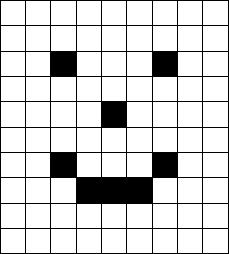# Numb3rs 319: Pandora's Box

The team investigates a plane crash and the shooting of the forest ranger who discovered the crash. Charlie uses a deconvolution algorithm to identify a smudged fingerprint, allowing them to identify the technician who tampered with the plane's software.

## Convolutions

A convolution is means of creating a new function h from two other functions f and g. For discrete functions we write h(n)=(f *g)(n)=&Sigmaif(i)g(i-n). Usually convolutions involve functions that take on non-zero values a finite number of times, since the sum would often be undefined otherwise. For such functions the the convolution evaluated at n tells us how much f overlaps with the translation of g by n. Convolutions can also be done with continuous functions, where we trade the sum for an integral. In this case h(x)=(f *g)(x)=&int-&infin&infin f(t)g(t-x) dt.

Activity 1
Calculate the following convolutions for f(n)=n2 for 1 &le n &le 4 and 0 otherwise, and g(n)=n for -2 &le n &le 2 and 0 otherwise.
1. 1. f *g
2. 2. g *f
3. 3. (2f) *g
4. 4. f *(2g)

The exercise hints at two basic properties of convolutions: they are commutative and associative with scalar multiplication. Convolutions are also associative, and the addition of functions distributes over convolutions.

Convolutions are frequently used in image processing. This is done by letting f represent the image data and letting g take on non-zero values only in a square centered at the origin. A square matrix is then used to represent g. For instance, the image below is shown before and after convolution with the matrix given below.
 -1 -1 -1 -1 8 -1 -1 -1 -1You can see that this convolution brought out the edges in the image. Convolutions similar to this are often called edge detectors. Other convolutions can do things like sharpen the image, blur it, or translate it. You can experiment with different convolutions using the applet here.

Activity 2: Try to figure out why the above matrix has the effect it does.
Activity 3: In the image below treat each square as a point in space with the bottom left square being the origin and the upper right square being (8,8).Treat a white square as having value 0 and a black square as having some non-zero value(thus adding the value of two black squares will give you a black square). You should also assume that any square not shown below is white. Consider the matrix below.
 0 0 1 0 0 0 1 0 0
Can you guess what will happen when this matrix ia convoluted with the image? Check your guess.

### Deconvolutions

The Fourier transform is another frequently used tool in signal processing. The Fourier transform takes a function and then produces a new one. If we view the original function as describing how a signal evolves over time the Fourier transform expresses that signal as a function of different frequency signals. For instance, a cosine function with frequency a has a Fourier transform that is zero everywhere except at a and -a.

Deconvolution involves finding f when we know g and h. This is a frequent problem in signal processing where h is the information we recieve from a device(like the Hubble telescope alluded to in the episode) and g is a distortion introduced to the original signal f. Often the exact form of gis unknown, but we know what kind of distortion it is. For example, if you look at a blurry picture you can often tell that the camera was moved while the picture was being taken, but you might not be able to tell exactly how much it was moved or in what direction. Finding the original signal is very difficult, and is usually done using one of several varieties of computer algorithms.

Activity 4: Throughout this activity we will assume the functions are discrete. We will try to find some deconvolutions
1. Let h be 1 for n = -1, 0, and 1, and 0 elsewhere. Let g be 0 everywhere except at the origin where it is 1. Find f such that h(n)=(f *g)(n). What does this tell you about the existence of an identity function for discrete convolutions?
2. Let h and g be as above except that g(1)=-1. You won't be able to find f, but see what you can figure out about it (Hint: You should find that most of f is constant).